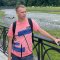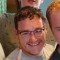# Defining the Database and TablesLast updated Sep 24th, 2021 | Page history | Improve this page | Report an issue

Let's say we have a Package called 'Storefinder'. We want to create a custom schema for that package. First off, we'll create a schema file with this name:

storefinder.mysql.schema.xml

If you note, we added in the 'mysql' postfix to the filename, since xPDO will eventually allow for multiple database development. We want to specify that this schema is for a MySQL table.

## Starting with a Database¶

Our current XML file looks like this:

``````<?xml version="1.0" encoding="UTF-8"?>
<model package="storefinder"
baseClass="xPDOObject"
platform="mysql"
defaultEngine="MyISAM"
tablePrefix="sf_"
phpdoc-package="storefinder"
phpdoc-subpackage="model"
version="1.1">
``````

First we'll tell the browser and parser that this is XML code with a standard XML header. Next, we're going to create a "model" tag, and put some attributes into it. The "model" tag is a representation of the database itself. The attributes are:

• package - The name of the xPDO package (note this is different than a "transport package", a Revolution term). This is how xPDO separates different models and manages them. Note: in the XML, the package name must be all lowercase.
• baseClass - This is the base class from which all your class definitions will extend. Unless you're planning on creating a custom xPDOObject extension, it's best to leave it at the default.
• platform — The database platform PDO driver you are using. At this time, xPDO supports mysql, sqlite, and sqlsrv PDO drivers. (Note: sqlsrv is no longer supported in MODX3.)
• defaultEngine — The default engine of the database tables, usually either MyISAM or InnoDB. xPDO recommends using MyISAM.
• tablePrefix — An optional parameter that can be set to override the default runtime table prefix. Useful for creating 3rd party components, but typically recommended to NOT define so the tablePrefix inherits from the MODX installation.
• phpdoc-package & phpdoc-subpackage — These are custom attributes we're going to use in our map and class files. They're not standard xPDO attributes, but show that you can put whatever you want as attributes.
• version — The version of the xPDO schema. As changes are made to the schema format, the version is updated to differentiate how the model is handled at runtime.

## Schema Versions¶

There are different schema versions. Between version 1.0 and 1.1, the biggest difference is how object indexes are defined. See Upgrading Models to Schema Version 1.1 for information on migrating your index definitions to the new format. Do not add version="1.1" (leave off the version attribute or set it to 1.0) if you have not yet described your indexes in the 1.1 schema format or xPDO will create the tables with no indexes.

## Defining Tables¶

Great! Now we've got our model definition. Let's add a table tag as the next line.

``````<object class="sfStore" table="stores" extends="xPDOSimpleObject">
``````

"Object" is our representation of a table, which will generate into an xPDOObject class when we're through. There are some attributes to note here:

• class — This is the name of the class we want to be generated from the table. Here, we'll use "sfStore". Note that instead of just "Store", we prefixed it with "sf" to prevent collisions with any other packages we might install that might also have Store tables.
• table — This should point to the actual database table name, minus the tablePrefix we specified for the package.
• extends — This is the class that it extends. Note that you can make subclasses and extended classes straight from the XML. Extended classes will inherit their parent class's fields.

You'll see here that this table extends "xPDOSimpleObject", rather than xPDOObject. This means that the table comes already with an "id" field, that is an auto-increment primary key.

Now that we've got a table definition for our stores table, let's add some field definitions to it:

``````<field key="name" dbtype="varchar" precision="100" phptype="string" null="false" default="" />
<field key="address" dbtype="varchar" precision="255" phptype="string" null="false" default="" />
<field key="city" dbtype="varchar" precision="255" phptype="string" null="false" default="" />
<field key="state" dbtype="varchar" precision="255" phptype="string" null="false" default="" />
<field key="zip" dbtype="varchar" precision="10" phptype="string" null="false" default="0" />
<field key="country" dbtype="varchar" precision="20" phptype="string" null="false" default="" />
<field key="phone" dbtype="varchar" precision="20" phptype="string" null="false" default="" />
<field key="fax" dbtype="varchar" precision="20" phptype="string" null="false" default="" />
<field key="active" dbtype="int" precision="1" attributes="unsigned" phptype="integer" null="false" default="0" />
``````

As you can see here, each column in our table has a field definition tag. From there, we have attribute properties for each field. Most of these are optional, depending on the database type of the column. Some of those attribute properties are:

• key — The key name of the column.
• dbtype — The DB type - such as varchar, int, text, tinyint, etc.
• precision — The precision of the field. Usually this is the max number of characters.
• attributes — Only applies to some DB types; in integers you can set to "unsigned" to make sure that the value is always positive.
• phptype — The corresponding PHP type of the DB field type.
• null — If the field can be NULL or not.
• default — The default starting value of the field should none be set.
• index (deprecated) — An optional field, when set, will add a type of index to the field. Some of the values are "pk", "index", and "fk". The index attribute is deprecated for Schema Version 1.1 and ignored when you generate your models as version 1.1. This is only valid for models with no version attribute (or the version set explicitly to 1.0).

Next, we'll define the indexes (conform schema version 1.1) that we want our table to have:

``````<index alias="name" name="name" primary="false" unique="false" type="BTREE">
<column key="name" length="" collation="A" null="false" />
</index>
<index alias="zip" name="zip" primary="false" unique="false" type="BTREE">
<column key="zip" length="" collation="A" null="false" />
</index>
``````

### The alias element¶

New in xPDO 2.2 is the ability to define field aliases. This can be useful when changing table structures to maintain backwards compatibility, or for defining useful aliases for the object API without having to modify table structure. The syntax is simple with two attributes, key (the alias) and field (the target field definition).

Now let's define an alias for the zip column called postalcode so the value can be accessed by either key:

``````<alias key="postalcode" field="zip" />
``````

And finally, we'll finish the table definition by closing the object tag:

``````</object>
``````

Now let's add an "sfOwner" class, which will represent any owners we have:

``````<object class="sfOwner" table="owners" extends="xPDOSimpleObject">
<field key="name" dbtype="varchar" precision="100" phptype="string" null="false" default="" index="index" />
<field key="email" dbtype="varchar" precision="255" phptype="string" null="false" default="" />

<index alias="name" name="name" primary="false" unique="false" type="BTREE">
<column key="name" length="" collation="A" null="false" />
</index>
</object>
``````

And since we want our stores to possibly have multiple owners, let's add a sfStoreOwner class, that will bridge the many-to-many relationship:

``````<object class="sfStoreOwner" table="store_owners" extends="xPDOSimpleObject">
<field key="store" dbtype="int" precision="10" attributes="unsigned" phptype="integer" null="false" default="0" index="index" />
<field key="owner" dbtype="int" precision="10" attributes="unsigned" phptype="integer" null="false" default="0" index="index" />

<index alias="store" name="store" primary="false" unique="false" type="BTREE">
<column key="store" length="" collation="A" null="false" />
</index>
<index alias="owner" name="owner" primary="false" unique="false" type="BTREE">
<column key="owner" length="" collation="A" null="false" />
</index>
</object>
``````

Let's close the model definition:

``````</model>
``````

We have a completed XML schema for our model. Now we'll need to define relationships for that schema.